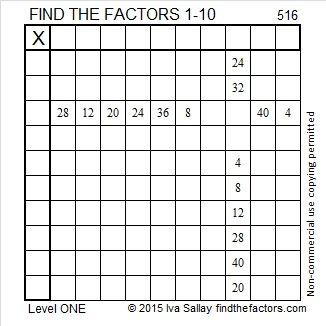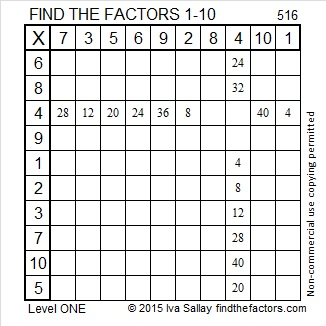# 516 Is an Untouchable Number

Look at this chart of the sum of the factors for the numbers 1 – 25:If we made the chart infinitely long using every counting number as n, there are certain numbers like 2, 5, 52, 88, and 96 that will NEVER appear in either column C or column D. Those numbers are called untouchable numbers, and 516 is one of them. Even though there are relatively few untouchable numbers, Paul Erdős proved that there are infinitely many of them.Print the puzzles or type the solution on this excel file: 10 Factors 2015-06-08

—————————————————————————————————

• 516 is a composite number.
• Prime factorization: 516 = 2 x 2 x 3 x 43, which can be written 516 = (2^2) x 3 x 43
• The exponents in the prime factorization are 2, 1, and 1. Adding one to each and multiplying we get (2 + 1)(1 + 1)(1 + 1) = 3 x 2 x 2 = 12. Therefore 516 has exactly 12 factors.
• Factors of 516: 1, 2, 3, 4, 6, 12, 43, 86, 129, 172, 258, 516
• Factor pairs: 516 = 1 x 516, 2 x 258, 3 x 172, 4 x 129, 6 x 86, or 12 x 43
• Taking the factor pair with the largest square number factor, we get √516 = (√4)(√129) = 2√129 ≈ 22.715633382—————————————————————————————————This site uses Akismet to reduce spam. Learn how your comment data is processed.